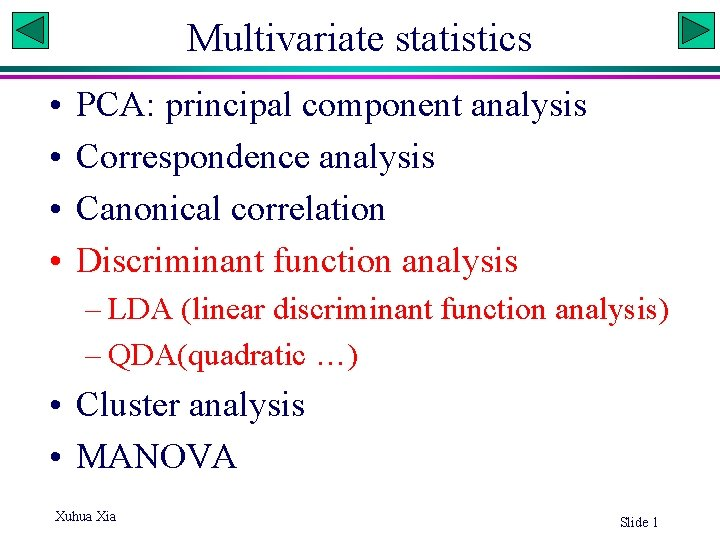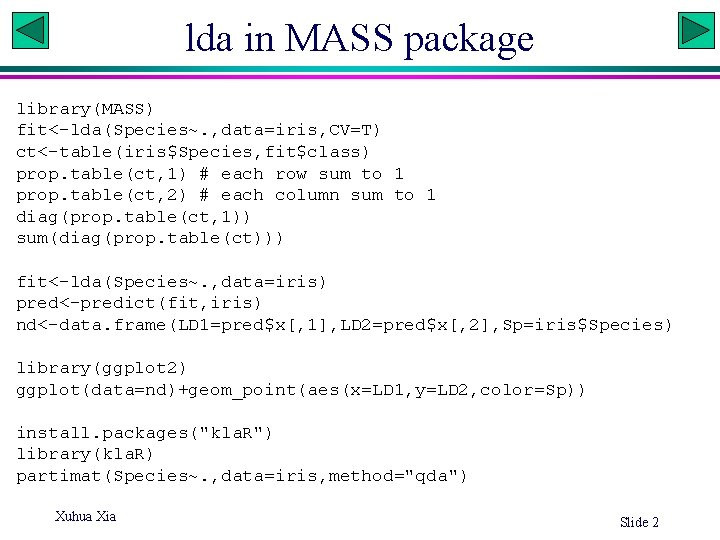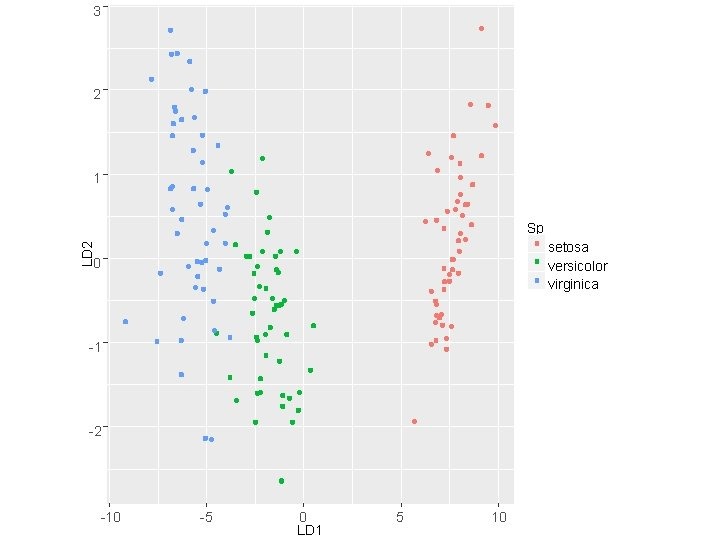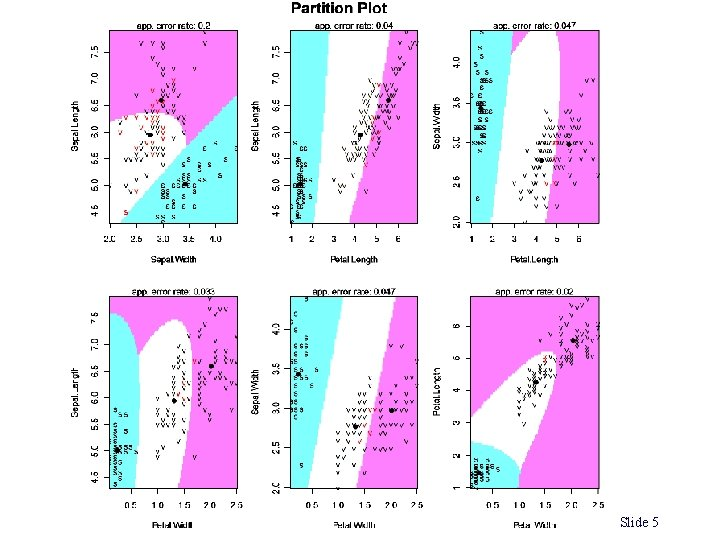# Multivariate statistics PCA principal component analysis Correspondence analysis

• Slides: 6Multivariate statistics • • PCA: principal component analysis Correspondence analysis Canonical correlation Discriminant function analysis – LDA (linear discriminant function analysis) – QDA(quadratic …) • Cluster analysis • MANOVA Xuhua Xia Slide 1lda in MASS package library(MASS) fit<-lda(Species~. , data=iris, CV=T) ct<-table(iris\$Species, fit\$class) prop. table(ct, 1) # each row sum to 1 prop. table(ct, 2) # each column sum to 1 diag(prop. table(ct, 1)) sum(diag(prop. table(ct))) fit<-lda(Species~. , data=iris) pred<-predict(fit, iris) nd<-data. frame(LD 1=pred\$x[, 1], LD 2=pred\$x[, 2], Sp=iris\$Species) library(ggplot 2) ggplot(data=nd)+geom_point(aes(x=LD 1, y=LD 2, color=Sp)) install. packages("kla. R") library(kla. R) partimat(Species~. , data=iris, method="qda") Xuhua Xia Slide 23 2 1 Sp LD 2 setosa versicolor virginica 0 -1 -2 -10 -5 0 LD 1 5 10qda in MASS package library(MASS) fit<-qda(Species~. , data=iris, CV=T) fit 2<-qda(Species~. , data=iris, CV=T, method="mle") ct<-table(iris\$Species, fit 2\$class) diag(prop. table(ct, 1)) sum(diag(prop. table(ct))) library(kla. R) partimat(Species~. , data=iris, method="qda") Xuhua Xia Slide 4Slide 5Cross-validation • Holdout method with jackknifing • K-fold cross validation: Divide data in each category into K sets. Each set will be used a test set and the rest will be pooled as training data. Every data point gets to be in a test set exactly once, and gets to be in a training set k-1 times. • Leave-one-out cross validation: K-fold cross validation taken to its extreme, with K equal to N, the number of data points in the set. Xuhua Xia Slide 6